Titanic survival classification

Current events on this problem
Keywords
titanic_starting_kit

# Paris Saclay Center for Data ScienceÂ¶

## Titanic RAMP: survival prediction of Titanic passengersÂ¶

Benoit Playe (Institut Curie/Mines ParisTech), Chloé-Agathe Azencott (Institut Curie/Mines ParisTech), Alex Gramfort (LTCI/Télécom ParisTech), Balázs Kégl (LAL/CNRS)

## IntroductionÂ¶

This is an initiation project to introduce RAMP and get you to know how it works.

The goal is to develop prediction models able to identify people who survived from the sinking of the Titanic, based on gender, age, and ticketing information.

The data we will manipulate is from the Titanic kaggle challenge.

### RequirementsÂ¶

• numpy>=1.10.0
• matplotlib>=1.5.0
• pandas>=0.19.0
• scikit-learn>=0.17 (different syntaxes for v0.17 and v0.18)
• seaborn>=0.7.1
In :
%matplotlib inline
import os
import glob
import numpy as np
from scipy import io
import matplotlib.pyplot as plt
import pandas as pd


## Exploratory data analysisÂ¶

### Loading the dataÂ¶

In :
train_filename = 'data/train.csv'
data = pd.read_csv(train_filename)
y_df = data['Survived']
X_df = data.drop(['Survived', 'PassengerId'], axis=1)
X_df.head(5)

Out:
Pclass Name Sex Age SibSp Parch Ticket Fare Cabin Embarked
0 3 Palsson, Mrs. Nils (Alma Cornelia Berglund) female 29.0 0 4 349909 21.075 NaN S
1 2 Beane, Mr. Edward male 32.0 1 0 2908 26.000 NaN S
2 3 Palsson, Miss. Stina Viola female 3.0 3 1 349909 21.075 NaN S
3 3 Torber, Mr. Ernst William male 44.0 0 0 364511 8.050 NaN S
4 2 Bystrom, Mrs. (Karolina) female 42.0 0 0 236852 13.000 NaN S
In :
data.describe()

Out:
PassengerId Survived Pclass Age SibSp Parch Fare
count 356.000000 356.000000 356.000000 290.000000 356.000000 356.000000 356.000000
mean 451.713483 0.384831 2.300562 29.123862 0.550562 0.412921 31.657970
std 260.505039 0.487240 0.833861 14.103122 1.120978 0.798415 43.474154
min 7.000000 0.000000 1.000000 0.920000 0.000000 0.000000 0.000000
25% 229.750000 0.000000 2.000000 19.000000 0.000000 0.000000 7.925000
50% 445.000000 0.000000 3.000000 28.000000 0.000000 0.000000 15.245800
75% 686.750000 1.000000 3.000000 37.000000 1.000000 1.000000 31.275000
max 890.000000 1.000000 3.000000 71.000000 8.000000 6.000000 263.000000
In :
data.count()

Out:
PassengerId    569
Survived       569
Pclass         569
Name           569
Sex            569
Age            448
SibSp          569
Parch          569
Ticket         569
Fare           569
Cabin          132
Embarked       567
dtype: int64

The original training data frame has 891 rows. In the starting kit, we give you a subset of 445 rows. Some passengers have missing information: in particular Age and Cabin info can be missing. The meaning of the columns is explained on the challenge website:

### Predicting survivalÂ¶

The goal is to predict whether a passenger has survived from other known attributes. Let us group the data according to the Survived columns:

In :
data.groupby('Survived').count()

Out:
PassengerId Pclass Name Sex Age SibSp Parch Ticket Fare Cabin Embarked
Survived
0 345 345 345 345 259 345 345 345 345 46 345
1 224 224 224 224 189 224 224 224 224 86 222

About two thirds of the passengers perished in the event. A dummy classifier that systematically returns "0" would have an accuracy of 62%, higher than that of a random model.

### Some plotsÂ¶

#### Features densities and co-evolutionÂ¶

A scatterplot matrix allows us to visualize:

• on the diagonal, the density estimation for each feature
• on each of the off-diagonal plots, a scatterplot between two features. Each dot represents an instance.
In :
from pandas.tools.plotting import scatter_matrix
scatter_matrix(data.get(['Fare', 'Pclass', 'Age']), alpha=0.2,
figsize=(8, 8), diagonal='kde');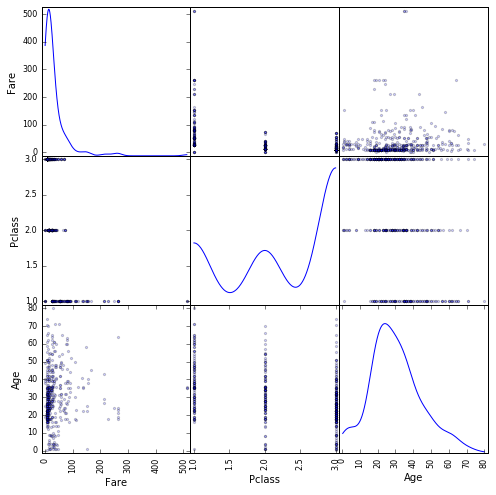#### Non-linearly transformed dataÂ¶

The Fare variable has a very heavy tail. We can log-transform it.

In :
data_plot = data.get(['Age', 'Survived'])
data_plot = data.assign(LogFare=lambda x : np.log(x.Fare + 10.))
scatter_matrix(data_plot.get(['Age', 'LogFare']), alpha=0.2, figsize=(8, 8), diagonal='kde');

data_plot.plot(kind='scatter', x='Age', y='LogFare', c='Survived', s=50, cmap=plt.cm.Paired);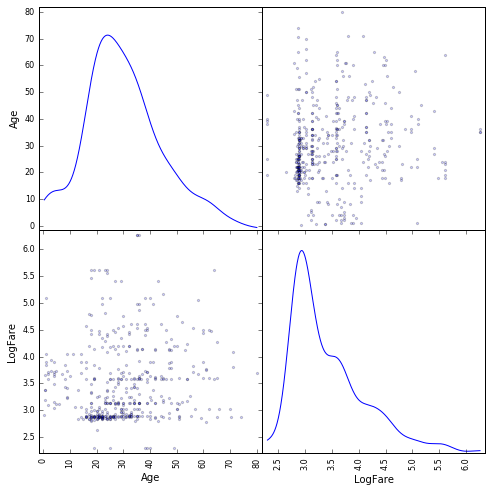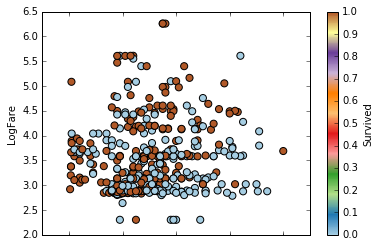#### Plot the bivariate distributions and marginals of two variablesÂ¶

Another way of visualizing relationships between variables is to plot their bivariate distributions.

In :
import seaborn as sns

sns.set()
sns.set_style("whitegrid")
sns.jointplot(data_plot.Age[data_plot.Survived == 1],
data_plot.LogFare[data_plot.Survived == 1],
kind="kde", size=7, space=0, color="b");

sns.jointplot(data_plot.Age[data_plot.Survived == 0],
data_plot.LogFare[data_plot.Survived == 0],
kind="kde", size=7, space=0, color="y");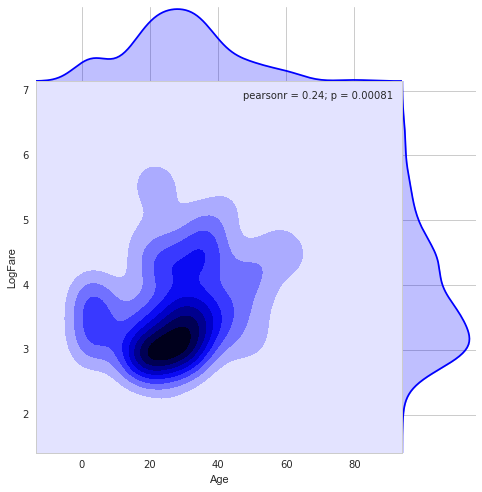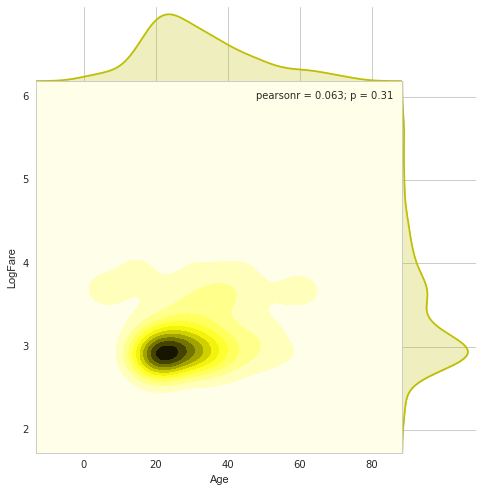## The pipelineÂ¶

For submitting at the RAMP site, you will have to write two classes, saved in two different files:

• the class FeatureExtractor, which will be used to extract features for classification from the dataset and produce a numpy array of size (number of samples $\times$ number of features).
• a class Classifier to predict survival

### Feature extractorÂ¶

The feature extractor implements a transform member function. It is saved in the file submissions/starting_kit/feature_extractor.py. It receives the pandas dataframe X_df defined at the beginning of the notebook. It should produce a numpy array representing the extracted features, which will then be used for the classification.

Note that the following code cells are not executed in the notebook. The notebook saves their contents in the file specified in the first line of the cell, so you can edit your submission before running the local test below and submitting it at the RAMP site.

In :
%%file submissions/starting_kit/feature_extractor.py
import pandas as pd

class FeatureExtractor():
def __init__(self):
pass

def fit(self, X_df, y):
pass

def transform(self, X_df):
X_df_new = pd.concat(
[X_df.get(['Fare', 'Age', 'SibSp', 'Parch']),
pd.get_dummies(X_df.Sex, prefix='Sex', drop_first=True),
pd.get_dummies(X_df.Pclass, prefix='Pclass', drop_first=True),
pd.get_dummies(
X_df.Embarked, prefix='Embarked', drop_first=True)],
axis=1)
X_df_new = X_df_new.fillna(-1)
XX = X_df_new.values
return XX

Overwriting submissions/starting_kit/feature_extractor.py


### ClassifierÂ¶

The classifier follows a classical scikit-learn classifier template. It should be saved in the file submissions/starting_kit/classifier.py. In its simplest form it takes a scikit-learn pipeline, assigns it to self.clf in __init__, then calls its fit and predict_proba functions in the corresponding member funtions.

In :
%%file submissions/starting_kit/classifier.py
from sklearn.linear_model import LogisticRegression
from sklearn.preprocessing import Imputer
from sklearn.pipeline import Pipeline
from sklearn.base import BaseEstimator

class Classifier(BaseEstimator):
def __init__(self):
self.clf = Pipeline([
('imputer', Imputer(strategy='median')),
('classifier', LogisticRegression(C=1.))
])

def fit(self, X, y):
self.clf.fit(X, y)

def predict_proba(self, X):
return self.clf.predict_proba(X)

Overwriting submissions/starting_kit/classifier.py


## Local testing (before submission)Â¶

It is important that you test your submission files before submitting them. For this we provide a unit test. Note that the test runs on your files in submissions/starting_kit, not on the classes defined in the cells of this notebook.

First pip install ramp-workflow or install it from the github repo. Make sure that the python files classifier.py and feature_extractor.py are in the submissions/starting_kit folder, and the data train.csv and test.csv are in data. Then run

ramp_test_submission

If it runs and print training and test errors on each fold, then you can submit the code.

In :
!ramp_test_submission

Testing Titanic survival classification
Reading train and test files from ./data ...
Reading cv ...
Training ./submissions/starting_kit ...
CV fold 0
train auc = 0.84
valid auc = 0.89
test auc = 0.83
train acc = 0.8
valid acc = 0.85
test acc = 0.76
train nll = 0.46
valid nll = 0.39
test nll = 0.5
CV fold 1
train auc = 0.85
valid auc = 0.86
test auc = 0.83
train acc = 0.81
valid acc = 0.8
test acc = 0.76
train nll = 0.44
valid nll = 0.45
test nll = 0.49
CV fold 2
train auc = 0.85
valid auc = 0.83
test auc = 0.82
train acc = 0.81
valid acc = 0.79
test acc = 0.76
train nll = 0.44
valid nll = 0.47
test nll = 0.5
CV fold 3
train auc = 0.84
valid auc = 0.91
test auc = 0.83
train acc = 0.8
valid acc = 0.82
test acc = 0.76
train nll = 0.46
valid nll = 0.4
test nll = 0.5
CV fold 4
train auc = 0.85
valid auc = 0.87
test auc = 0.83
train acc = 0.81
valid acc = 0.81
test acc = 0.76
train nll = 0.45
valid nll = 0.44
test nll = 0.51
CV fold 5
train auc = 0.84
valid auc = 0.89
test auc = 0.84
train acc = 0.8
valid acc = 0.81
test acc = 0.76
train nll = 0.45
valid nll = 0.43
test nll = 0.5
CV fold 6
train auc = 0.84
valid auc = 0.88
test auc = 0.84
train acc = 0.81
valid acc = 0.79
test acc = 0.76
train nll = 0.45
valid nll = 0.45
test nll = 0.51
CV fold 7
train auc = 0.85
valid auc = 0.86
test auc = 0.84
train acc = 0.81
valid acc = 0.79
test acc = 0.76
train nll = 0.45
valid nll = 0.45
test nll = 0.5
----------------------------
train auc = 0.85 ± 0.005
train acc = 0.81 ± 0.006
train nll = 0.45 ± 0.007
valid auc = 0.87 ± 0.023
valid acc = 0.81 ± 0.02
valid nll = 0.44 ± 0.024
test auc = 0.83 ± 0.006
test acc = 0.76 ± 0.003
test nll = 0.5 ± 0.005


## Submitting to ramp.studioÂ¶

Once you found a good feature extractor and classifier, you can submit them to ramp.studio. First, if it is your first time using RAMP, sign up, otherwise log in. Then find an open event on the particular problem, for example, the event titanic for this RAMP. Sign up for the event. Both signups are controled by RAMP administrators, so there can be a delay between asking for signup and being able to submit.

Once your signup request is accepted, you can go to your sandbox and copy-paste (or upload) feature_extractor.py and classifier.py from submissions/starting_kit. Save it, rename it, then submit it. The submission is trained and tested on our backend in the same way as ramp_test_submission does it locally. While your submission is waiting in the queue and being trained, you can find it in the "New submissions (pending training)" table in my submissions. Once it is trained, you get a mail, and your submission shows up on the public leaderboard. If there is an error (despite having tested your submission locally with ramp_test_submission), it will show up in the "Failed submissions" table in my submissions. You can click on the error to see part of the trace.

After submission, do not forget to give credits to the previous submissions you reused or integrated into your submission.

The data set we use at the backend is usually different from what you find in the starting kit, so the score may be different.

The usual way to work with RAMP is to explore solutions, add feature transformations, select models, perhaps do some AutoML/hyperopt, etc., locally, and checking them with ramp_test_submission. The script prints mean cross-validation scores

----------------------------
train auc = 0.85 ± 0.005
train acc = 0.81 ± 0.006
train nll = 0.45 ± 0.007
valid auc = 0.87 ± 0.023
valid acc = 0.81 ± 0.02
valid nll = 0.44 ± 0.024
test auc = 0.83 ± 0.006
test acc = 0.76 ± 0.003
test nll = 0.5 ± 0.005

The official score in this RAMP (the first score column after "historical contributivity" on the leaderboard) is area under the roc curve ("auc"), so the line that is relevant in the output of ramp_test_submission is valid auc = 0.87 ± 0.023. When the score is good enough, you can submit it at the RAMP.

## Other models in the starting kitÂ¶

You can also keep several other submissions in your work directory submissions. random_forest_20_5 uses the same feature extractor as starting_kit but another classifier.

In :
%%file submissions/random_forest_20_5/feature_extractor.py
import pandas as pd

class FeatureExtractor():
def __init__(self):
pass

def fit(self, X_df, y):
pass

def transform(self, X_df):
X_df_new = pd.concat(
[X_df.get(['Fare', 'Age', 'SibSp', 'Parch']),
pd.get_dummies(X_df.Sex, prefix='Sex', drop_first=True),
pd.get_dummies(X_df.Pclass, prefix='Pclass', drop_first=True),
pd.get_dummies(
X_df.Embarked, prefix='Embarked', drop_first=True)],
axis=1)
X_df_new = X_df_new.fillna(-1)
XX = X_df_new.values
return XX

Overwriting submissions/random_forest_20_5/feature_extractor.py

In :
%%file submissions/random_forest_20_5/classifier.py
from sklearn.linear_model import LogisticRegression
from sklearn.preprocessing import Imputer
from sklearn.pipeline import Pipeline
from sklearn.base import BaseEstimator

class Classifier(BaseEstimator):
def __init__(self):
self.clf = Pipeline([
('imputer', Imputer(strategy='median')),
('classifier', LogisticRegression(C=1.0))
])

def fit(self, X, y):
self.clf.fit(X, y)

def predict_proba(self, X):
return self.clf.predict_proba(X)

Overwriting submissions/random_forest_20_5/classifier.py


You can train this by specifying the subfolder in the test script.

In :
!ramp_test_submission --submission random_forest_20_5

Testing Titanic survival classification
Reading train and test files from ./data ...
Reading cv ...
Training ./submissions/random_forest_20_5 ...
CV fold 0
train auc = 0.84
valid auc = 0.89
test auc = 0.83
train acc = 0.8
valid acc = 0.85
test acc = 0.76
train nll = 0.46
valid nll = 0.39
test nll = 0.5
CV fold 1
train auc = 0.85
valid auc = 0.86
test auc = 0.83
train acc = 0.81
valid acc = 0.8
test acc = 0.76
train nll = 0.44
valid nll = 0.45
test nll = 0.49
CV fold 2
train auc = 0.85
valid auc = 0.83
test auc = 0.82
train acc = 0.81
valid acc = 0.79
test acc = 0.76
train nll = 0.44
valid nll = 0.47
test nll = 0.5
CV fold 3
train auc = 0.84
valid auc = 0.91
test auc = 0.83
train acc = 0.8
valid acc = 0.82
test acc = 0.76
train nll = 0.46
valid nll = 0.4
test nll = 0.5
CV fold 4
train auc = 0.85
valid auc = 0.87
test auc = 0.83
train acc = 0.81
valid acc = 0.81
test acc = 0.76
train nll = 0.45
valid nll = 0.44
test nll = 0.51
CV fold 5
train auc = 0.84
valid auc = 0.89
test auc = 0.84
train acc = 0.8
valid acc = 0.81
test acc = 0.76
train nll = 0.45
valid nll = 0.43
test nll = 0.5
CV fold 6
train auc = 0.84
valid auc = 0.88
test auc = 0.84
train acc = 0.81
valid acc = 0.79
test acc = 0.76
train nll = 0.45
valid nll = 0.45
test nll = 0.51
CV fold 7
train auc = 0.85
valid auc = 0.86
test auc = 0.84
train acc = 0.81
valid acc = 0.79
test acc = 0.76
train nll = 0.45
valid nll = 0.45
test nll = 0.5
----------------------------
train auc = 0.85 ± 0.005
train acc = 0.81 ± 0.006
train nll = 0.45 ± 0.007
valid auc = 0.87 ± 0.023
valid acc = 0.81 ± 0.02
valid nll = 0.44 ± 0.024
test auc = 0.83 ± 0.006
test acc = 0.76 ± 0.003
test nll = 0.5 ± 0.005


## Working in the notebookÂ¶

When you are developing and debugging your submission, you may want to stay in the notebook and execute the workflow step by step. You can import problem.py and call the ingredients directly, or even deconstruct the code from ramp-workflow.

In :
import imp
problem = imp.load_source('', 'problem.py')


Get the training data.

In :
X_train, y_train = problem.get_train_data()


Get the first cv fold, creating training and validation indices.

In :
train_is, test_is = list(problem.get_cv(X_train, y_train))
test_is

Out:
array([319, 289, 567, 294, 112, 216, 186, 195, 478, 531, 451, 282,  43,
189, 177, 159, 342, 513, 514, 215, 431, 132, 139, 233, 136, 237,
111, 271, 354,  61, 427, 149, 523, 475, 376, 328, 419, 546,   4,
83,  75,   5, 516,  81, 301, 500, 562, 309, 318, 473, 307, 207,
346, 297, 404, 146, 119, 209, 158, 460,  34, 183, 131, 272,  21,
461, 125, 400, 138, 173, 247, 549, 334, 483, 432,   9, 316, 528,
193, 445, 167, 179, 252, 229, 517, 504, 364, 539,  82, 331, 181,
240,  26, 424, 457, 392, 150, 226,  27, 340, 230, 178, 446,   7,
47, 332, 208, 390, 557, 377, 256, 227, 262, 352])

Train your starting kit.

In :
fe, clf = problem.workflow.train_submission(
'submissions/starting_kit', X_train, y_train, train_is)


Get the full prediction (train and validation).

In :
y_pred = problem.workflow.test_submission((fe, clf), X_train)


Print the training and validation scores.

In :
score_function = problem.score_types


score_function is callable, wrapping scikit-learn's roc_auc_score. It expects a 0/1 vector as ground truth (since out labels are 0 and 1, y_train can be passed as is), and a 1D vector of predicted probabilities of class '1', which means we need the second column of y_pred.

In :
score_train = score_function(y_train[train_is], y_pred[:, 1][train_is])
print(score_train)

0.84298842199

In :
score_valid = score_function(y_train[test_is], y_pred[:, 1][test_is])
print(score_valid)

0.887278582931


You can check that it is just a wrapper of roc_auc_score.

In :
from sklearn.metrics import roc_auc_score
print(roc_auc_score(y_train[train_is], y_pred[:, 1][train_is]))

0.84298842199


Get the independent test data.

In :
X_test, y_test = problem.get_test_data()


Test the submission on it.

In :
y_test_pred = problem.workflow.test_submission((fe, clf), X_test)

In :
score_test = score_function(y_test, y_test_pred[:, 1])
print(score_test)

0.827582728006


If you want to execute training step by step, go to the feature_extractor_classifier, feature_extractor, and classifier workflows and deconstruct them.

First load the submission files and instantiate the feature extractor and regressor objects.

In :
feature_extractor = imp.load_source(
'', 'submissions/starting_kit/feature_extractor.py')
fe = feature_extractor.FeatureExtractor()
classifier = imp.load_source(
'', 'submissions/starting_kit/classifier.py')
clf = classifier.Classifier()


Select the training folds.

In :
X_train_train_df = X_train.iloc[train_is]
y_train_train = y_train[train_is]


Fit the feature extractor.

In :
fe.fit(X_train_train_df, y_train_train)


Transform the training dataframe into numpy array.

In :
X_train_train_array = fe.transform(X_train_train_df)


Fit the classifier.

In :
clf.fit(X_train_train_array, y_train_train)


Transform the whole (training + validation) dataframe into a numpy array and compute the prediction.

In :
X_train_array = fe.transform(X_train)
y_pred = clf.predict_proba(X_train_array)


Print the errors.

In :
score_train = score_function(y_train[train_is], y_pred[:, 1][train_is])
print(score_train)

0.84298842199

In :
score_valid = score_function(y_train[test_is], y_pred[:, 1][test_is])
print(score_valid)

0.887278582931


## More informationÂ¶

You can find more information in the README of the ramp-workflow library.

## ContactÂ¶

Don't hesitate to contact us.